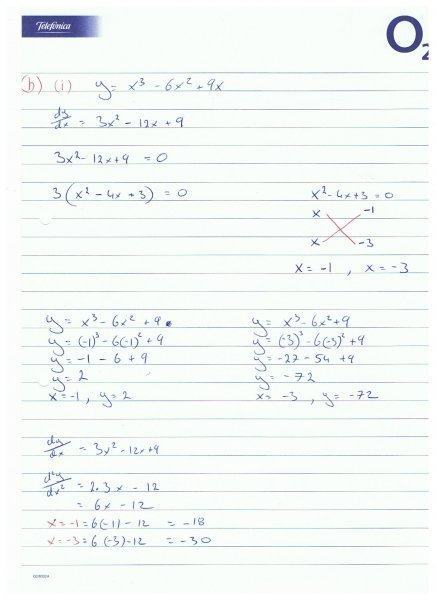# Solving turning points

• anthonyk2013

#### anthonyk2013I'm wondering if I'm right or wrong. question is Apply differentiation to determine the co-ordinates of the turning points on the graph Y=X3-6X2+9x
and finf max and min turning points

View attachment 77039 I'm wondering if I'm right or wrong. question is Apply differentiation to determine the co-ordinates of the turning points on the graph Y=X3-6X2+9x
and finf max and min turning points

When you are solving the equation x2 - 4x + 3 = 0, you made a silly mistake.

This equation has two factors, namely (x - 3) and (x - 1). Re-writing x2 - 4x + 3 = (x - 3)(x - 1) = 0. By setting each factor equal to zero independently, you can make the equation true; thus x - 3 = 0 or x - 1 = 0. The solutions you have, x = -3 and x = -1, are incorrect.

When you are solving the equation x2 - 4x + 3 = 0, you made a silly mistake.

This equation has two factors, namely (x - 3) and (x - 1). Re-writing x2 - 4x + 3 = (x - 3)(x - 1) = 0. By setting each factor equal to zero independently, you can make the equation true; thus x - 3 = 0 or x - 1 = 0. The solutions you have, x = -3 and x = -1, are incorrect.

Ok I did have trouble they're , I'm a long time away from this type of stuff and trying to remember from 25 years ago. Where should I go fro here so?

Correct the solutions you have and work from there. All of your work past this point must be checked again using the correct solutions.

I used quadratic formula and got x=1,x=3
sub in x=3
Y=x3-6x2+9
Y=(3)3-6(3)2+9
Y=27-54+9
Y=-18

sub in x=1
Y=(1)3-6(1)2+9
Y=1-6+9
Y=4

dy/dx=3x2-12x+9

d2y/dx2=6x-12

X=1, 6(1)-12=-6
X=3, 6(3)-12=6

Last edited: